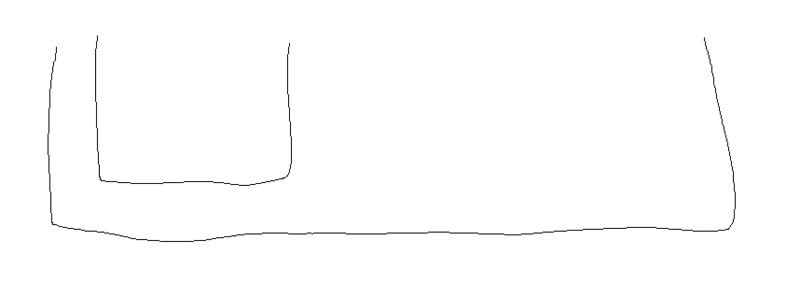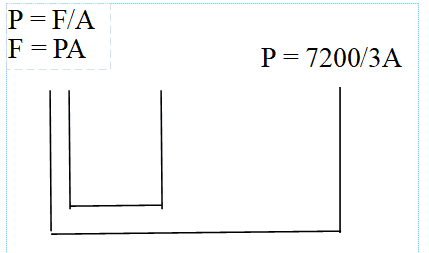# Pascal's Law Problems

## Homework Statement

The large cylinder in a hydraulic press has 3 times the surface area of the small cylinder. What force should be applied to the small cylinder to create a lifting force of 7200 Newtons

In a hydraulic-brake system, a force of 25 N can be applied to a surface area of 5 cm^2. What force can thus be exerted on each brake cylinder having an area of 100 cm^2

I have completed these problems but I am unsure if my work makes sense or if it is correct.

P = F/A

## The Attempt at a Solution

For the first problem, I identified the lifting force, 7200, as P (pressure). I thought that if the large cylinder is 3A (A is surface area), then that means
7200 = F/3A And since surface area is inversely proportional to the pressure, then the force is:
7200/3 = 2400 N

For the second problem, I applied the same formula to first answer for the pressure of the preliminary set of information I am given:
P = F/A
P = 25/5
P = 5
Then I used 500 again to find the force applied for the 100cm^2 cylinder
5 = F/A
5 = F/100
F = 500 Newtons

Are these solutions correct? I am unsure about the units I should be using also, because to me my answer for the second question seems wrong. But that was the only way that made sense to me.

Thanks.

In your solution, the lifting FORCE is not a PRESSURE. What is given as 7200 N is the force, not the pressure.

•Abu
In your solution, the lifting FORCE is not a PRESSURE. What is given as 7200 N is the force, not the pressure.
Thank you. I was also wondering that too because I thought pressure was Newton per meter squared, not simply Newtons. That makes more sense now..
Also, is the first problem saying that 7200 Newtons is also the force applied for the large cylinder? I am confused about the significance of the first sentence "The large cylinder in a hydraulic press has 3 times the surface area of the small cylinder".

Do you know how a hydraulic press works? Do you have a diagram?

Do you know how a hydraulic press works? Do you have a diagram?
Wow, I just had a moment of realization. I am almost sure I know how a hydraulic press works. I have a very crude diagram I just drew right now on Paint, but I know what it means now by small and large cylinder. I'll post another attempt of the problem in just a moment.#### Attachments

Do you know how a hydraulic press works? Do you have a diagram?
Okay so.. sorry for the delay, but I created a better diagramSo here is what I came up with: If the force applied is directly proportional with the surface area, then in order to create a lifting force of 7200 Newtons with 3 times the surface area, then the force applied must be 7200/3 which is 2400 Newtons.
If I did this correct, how is my solution for the second problem? Thank you.